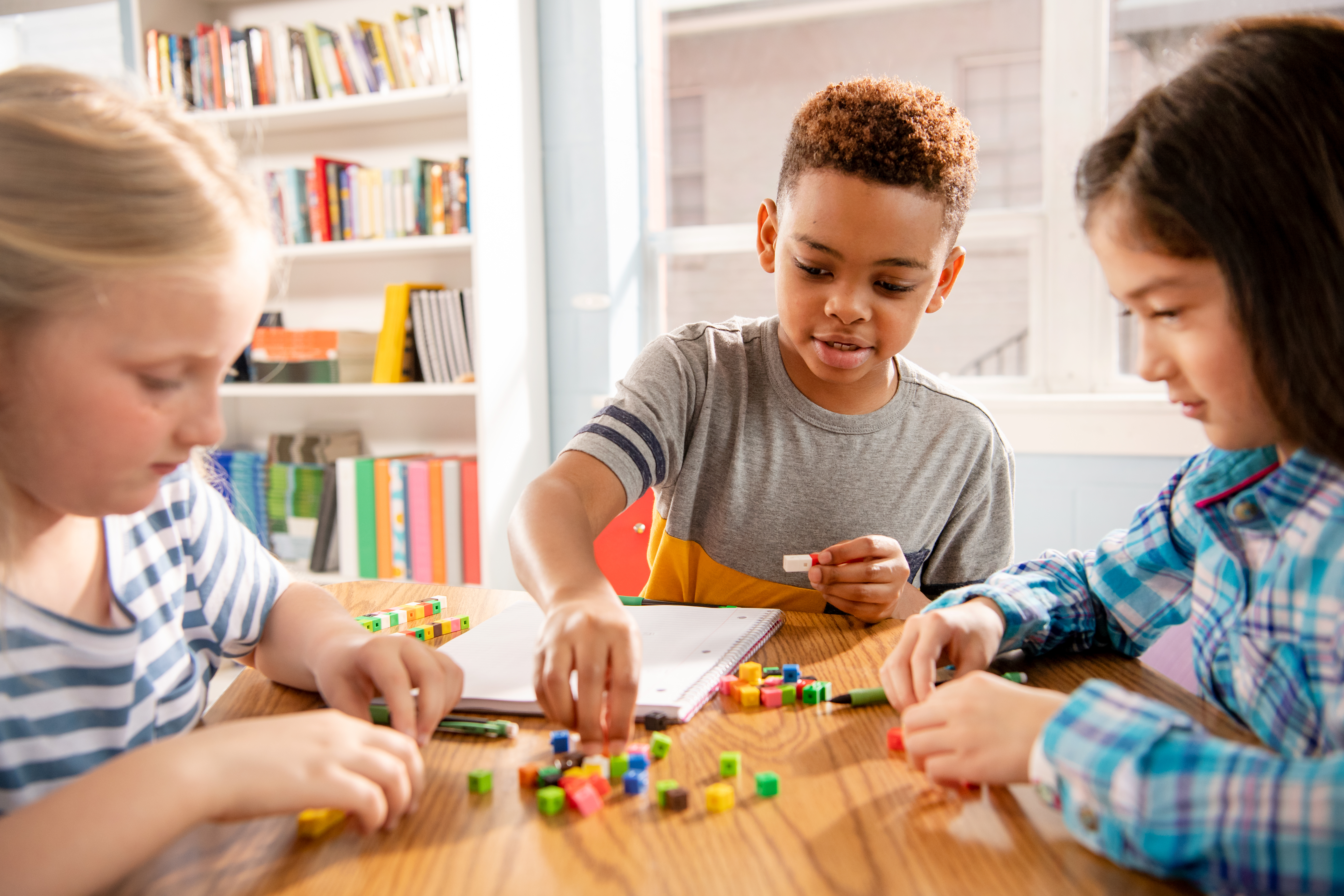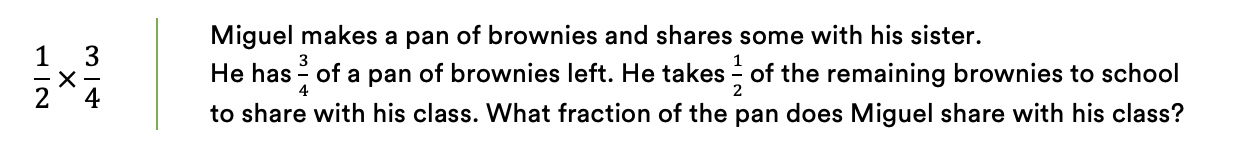Topics: Implementation Support

# Building Mathematical Thinkers, One Application Problem at a Time

November 11, 2020Posted in: Aha! Blog > Eureka Math Blog > Implementation Support > Building Mathematical Thinkers, One Application Problem at a Time

When am I ever going to use this in life?

My students asked me this question all the time. The truth is, I didn’t know what my students’ futures would be or what math they would need to use. We didn’t do math problems just so they would be able to do those same problems later in life. We did them so the students would learn to think mathematically and be able to solve the new problems they would encounter at each of life’s turns.

The Application Problems that we pose to students help them understand mathematical concepts by making the contexts more concrete. These problems are essential for students to build the skill to transfer mathematical learning to new and different contexts. That is the goal—educating students who know and can apply mathematical concepts to the world around them.

Help your students connect Application Problems and symbolic math.

Many of us learned how to solve math problems procedurally. For example, you probably learned to multiply fractions with the rule multiply the numerators, then multiply the denominators. But how many of us understood why we were doing that and when we needed to do it to solve a problem?

Consider this symbolic math problem and a related Application Problem.Connecting symbolic math to an Application Problem helps clarify the purpose and the meaning of the mathematics. Students who connect the fractions in the abstract problem to the actions and results in the Application Problem build a deeper understanding of fractions and of multiplication. Exposure to and discussion of connections to problems in additional contexts help students learn to appropriately transfer their knowledge about fraction multiplication to new situations.

Use the Read–Draw–Write framework to help your students solve Application Problems.

Eureka Math® emphasizes the Read–Draw–Write (RDW) process as an overarching framework to help students make sense of and solve Application Problems. This framework encourages students to make connections between the problem and the symbolic math they learn.

 Read Students read the entire problem to get an idea of the big picture. They consider what is known and what is unknown. Draw Students reread the problem, one chunk of information at a time, and stop to model their thinking with a math drawing. After each chunk, students ask themselves, Can I draw something now? What can I draw? Write Students write a symbolic math statement that represents their thinking, based on their math drawing. Next, they recontextualize the problem and write an answer statement in the original context of the problem. Finally, students consider the reasonableness of their answer.

The RDW process is a flexible approach that can be applied to any word problem students encounter. It encourages students to make sense of the mathematical information instead of scanning for numbers and keywords, which can often point students in the wrong direction. More information about the RDW process can be found in the Module 1 Overview at each grade level in Kindergarten through Grade 5.

Use different modes of delivery for different purposes.

You and your students may engage with Application Problems in different ways, including modeling, guided application, and independent practice.

 Systematic Modeling of and Instruction in the RDW Process The teacher models the entire RDW process with interactive questioning and some choral response. After students complete the problems, they might reflect with a partner on the steps they used to solve. Then students might be given the same or a similar problem to solve for homework. Guided Application of the RDW Process Each student has a copy of the question. Though guided by the teacher, students work independently at times and then come together again. Timing is important. The debrief might include selecting different student work to share. Independent Practice with Productive Struggle The students are given a word problem to solve and an extended amount of time to solve it. The teacher circulates, observes, lightly supports, and thinks about which student’s work to share. When sharing student work, the teacher uses questioning to encourage students to think about the work.

Think about how moving flexibly among these three modes of delivery can support students. Modeling is especially useful for situations where you might want students to try something new. Guided application might be more effective once students have some familiarity with the concepts or methods. With independent practice, students can engage in productive struggle when they have enough foundation to tackle the new aspects of the problem on their own. You will likely find yourself using a combination of these modes of delivery, depending on the math problem and your students’ needs. However, don’t neglect independent practice. Those moments of productive struggle can lead to the most student growth.

Eureka Math builds application throughout each topic and module instead of only addressing word problems in their own separate lesson. This allows students to gain experience with contextualizing and decontextualizing mathematics. The result is that your students will be more confident and flexible problem solvers. They will transfer their mathematical knowledge to new situations throughout the school year and develop the skills needed to independently solve new problems.

Topics: Implementation Support